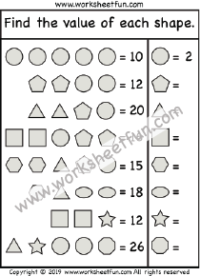Algebra Shape Variables – Solving Equations Puzzle – One Worksheet

Algebra Shape Variables – Solving Equations Puzzle

Find the value of each shape.Balancing Equations

Equations

Pan Balance Problems

Algebraic Reasoning